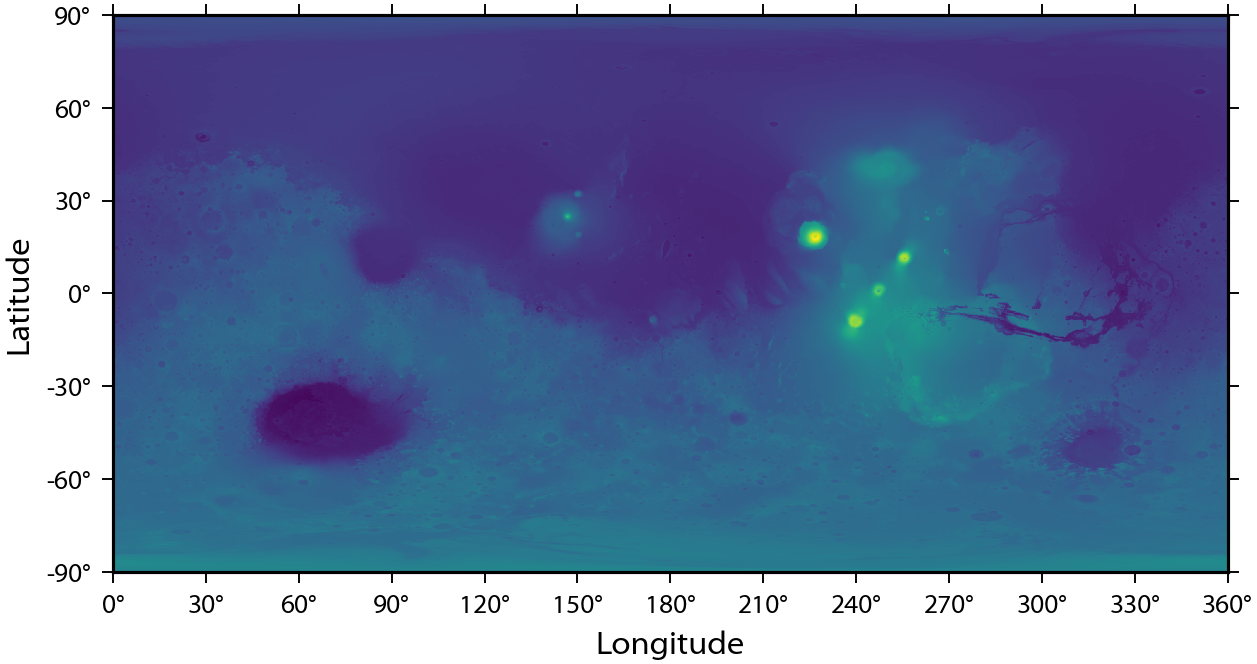# Plotting Maps in pyshtools¶

pyshtools allows for the creation of publication quality images of geographical data with a minimum of effort. A basic image can be created simply by using the plot() method of an SHGrid class instance, and a variety of customizations can be specified by adding human-readable arguments. In this notebook, we demonstrate the major features of this plotting routine, which include

1. Colormaps
2. Colorbars
3. Ticks and Labels
4. Map Projections
5. Creating Subplots

To demonstrate these features, we will make use of a few optional modules that will need to be installed. cartopy and pygmt allow for geographic projections, and palettable provides easy access to some useful scientific color maps.

In :
import pyshtools as pysh
import matplotlib.pyplot as plt

from cartopy import crs as ccrs
import pygmt
from palettable import scientific as scm

%config InlineBackend.figure_format = 'retina'  # if you are not using a retina display, comment this line!


For our example, we will make use of the spherical harmonic coefficients of the shape of Mars. We read the coefficients from file, divide by 1000 to convert to km, set both the degree 0 and flattening terms to zero, expand the coefficients on a grid, and then plot the grid using default options.

In :
clm = pysh.SHCoeffs.from_file('../ExampleDataFiles/MarsTopo719.shape') / 1000.
clm.coeffs[0,0,0] = 0.
clm.coeffs[0,2,0] = 0.
grid = clm.expand()

fig, ax = grid.plot(show=False)  # show=False is used only to avoid warnings when executing as a jupter notebookThe plot() method returns a matplotlib figure and its corresponding axes. When projecting data using pygmt, only a pygmt.Figure instance is returned.

## 1. Colormaps¶

The default colormap for pyshtools is 'viridis', which is both perceptually uniform and optimized for people with the most common form of color-vision deficiency. The first thing we can do is to select a different colormap. When using matplotlib colormaps, it is only necessary to specify the argument cmap and provide the name (as a string) of the colormap. For this example, we choose the matplotlib colormap 'RdBu' that goes from red to blue:

In :
fig, ax = grid.plot(cmap='RdBu',
show=False)